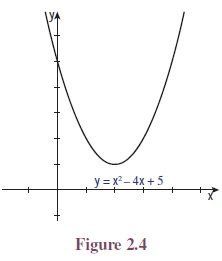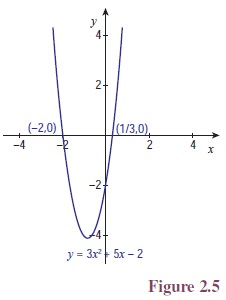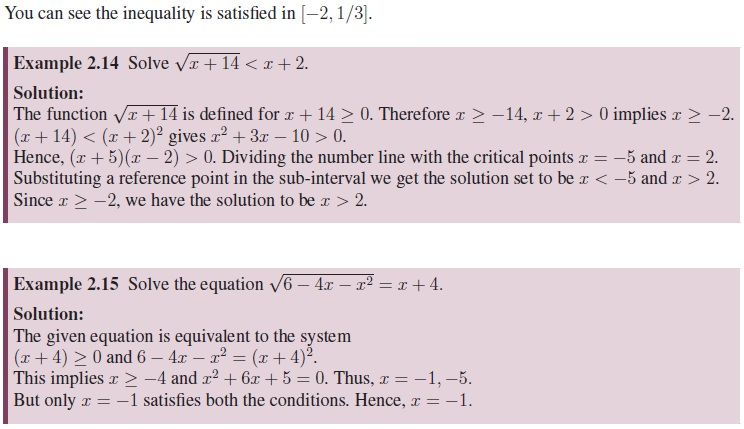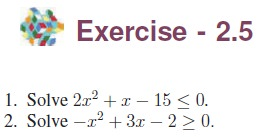Home | | Maths 11th std | Quadratic Functions

## Chapter: 11th Mathematics : UNIT 2 : Basic Algebra

Mathematics : Basic Algebra

In earlier classes we have learnt that for any z R and n N, zn = z · z · z · · · z (n-times).

A function of the form P (x) = ax2 + bx + c, where a, b, c R are constants and a ¹ 0, is called a quadratic function. If P (t) = 0 for some t R, then we say t is a zero of P (x).Here we shall learn to solve the quadratic inequalities ax2 + bx + c < 0 or ax2 + bx + c > 0.

i.            First solve ax2 + bx + c = 0.

ii.            If there are no real solutions, then one of the above inequality holds for all x R

iii.            If there are real solutions, which are called critical points, then label those points on the number line.

iv.            Note that these critical points divide the number line into disjoint intervals. (It is possible that there may be only one critical point.)

v.            Choose one representative number from each interval.

vi.            Substitute that these representative numbers in the inequality.

vii.            Identify the intervals where the inequality is satisfied.Tags : Definition, Formula, Solved Example Problems, Exercise | Algebra | Mathematics , 11th Mathematics : UNIT 2 : Basic Algebra
Study Material, Lecturing Notes, Assignment, Reference, Wiki description explanation, brief detail
11th Mathematics : UNIT 2 : Basic Algebra : Quadratic Functions | Definition, Formula, Solved Example Problems, Exercise | Algebra | Mathematics